# Introduction

Backpropagation might seem especially hard. If you search the internet , you can find many results including but not limited to photos that contain seemingly strange math symbols(if you do not know calculus). This is why I tried to write this article in order to shed light on the math behind backpropagation. Now, I am not sure if I have achieved my purpose to make the average reader understand more about this topic, but I hope that I have motivated him to learn more about this topic and neural networks in general.

(Example of an image that I found on internet after a quick search of backpropagation to show its complexity and its unfriendliness to the average reader, as in order to understand it requires knowledge of vector calculus)

In this article I will try to explain this essential process as plain as I can.

# Some knowledge needed before discussing backpropagation

I will speculate that you are familiar with what neural networks are (if not you can see this article in wikipedia or even this

introductory topic in devforum that provides example code of a neural net) but I will briefly discuss forward propagation for the shake of continuity.

# Forward Propagation

The neural network that we will be examining is the perceptron which is the simplest neural network, that consists of a single neuron, a n number of inputs and one output.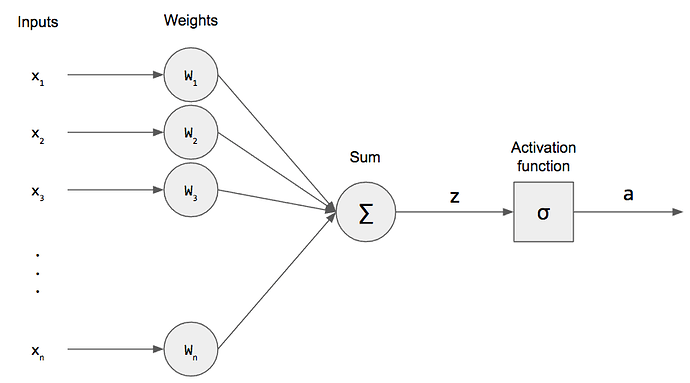(image 2) A perceptron

Forward propagation is the process of passing the data through the perceptron. There are three steps when the forward propagation is carried out in a perceptron.

# Step 1

We multiple each input (let’s say x1) with its corresponding weight (the w1) and sum all the multiplied values.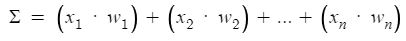Which can also be written as :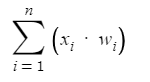# Step 2

We add a bias to the summation of the multiplied values . You can call the sum however you like, lets call it z.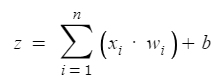# Step 3

We pass the value of z to an activation function . Some popular activation functions are the following:

(image 3 Some activation functions)

These are the three steps of forward propagation in a perceptron.

# Some other basic knowledge

What is the derivative?

Consider a function y = f(x).

We call the increase Δx of a variable x its change, as x increases or decreases from a value x = x0 to an other value x = x1. Therefore Δx = x1- x0, which can be written as x1 = x0 + Δx (Note: y1 = f(x1) = f(x0 + Δx)). If the variable x is changing by Δx from a value of x=x0( that is if x changes from x=x0 to x = x0 + Δx) then the function will change by Δy = f(x0 + Δx) - f(x0) from the value y = f(x0) (that is the same with saying y1 - y0=Δy or f(x1) - f(x0) = Δy ). The quotient Δy / Δx = Change in y/ Change in x is called the instantaneous rate of change of the function

The derivative of a function y=f(x) with respect to x is defined by the following: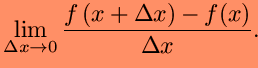The essence of calculus is the derivative . The derivative is the instantaneous rate of change of a function with respect to one of its variables. This is equivalent to finding the slope of the tangent line to the function at a point.

Because it is really time consuming to use the formula above every time we need to find the derivative of a function, there are luckily for us some formulas which we can use. An example of these is given in the following image:

Note that f’(x) and dy/dx(when we have an equation y=f(x)) symbolizes the derivative of a function f(x).

There are also some rules that we need to take into consideration when trying to find the derivative of a function.

For example if we want to find the derivative of a function y=f(u) and u = g(x) with respect to x then the derivative of the function y=f(u) with respect to x is the following: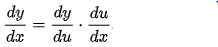This is known as the chain rule.

Other rules are the following:

# Back to backpropagation

Let’s start with our definition of Backpropagation

Backpropagation is an algorithm used to train the neural network of the chain rule method . In simple terms, after the data passes through the perceptron (forward propagation) , this algorithm does the backward pass to adjust the model’s parameters based on weights and biases.

Now, we will examine a really simple model of neural network in order to understand what Backpropagation really is. Consider a simple neural network consisting of two nodes on two layers, the input layer and the output layer. There is no bias unit.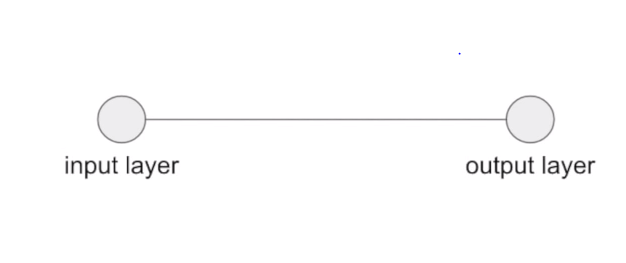We choose for activation function just the input multiplied by a randomly generated weight and hence the output is:

a = i * w Where i is the input, w the weight and a the output

Before training we initialize the weights : w = 0.8
For training we will use a training set which consists of a single input/ output pair:
input i = 1.5 , desired output y = 0.5

That means that with the value 0.8 we would like our network to produce the value of 4. With our current weight however, our network produces the value of a = i*w = 0.8 * 1.5 = 1.2
For the network to be able to train itself, we will need to find the error, which we will do using the MSE as our cost function :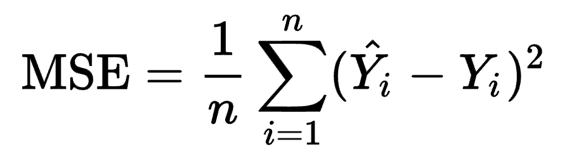Since n = 1 in our case our cost function becomes the following:

C = (ydot - y)^2 = (y-a)^2

Looking at the activation as a function of weight : a(w) = 1.5 * w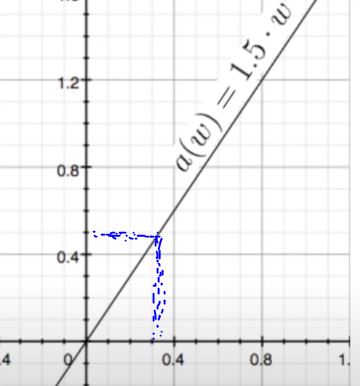we can see that in order a to be equal with 0.5(which is our desired output) w needs to be near 0.3.

By bringing the error function (y-a)^2 :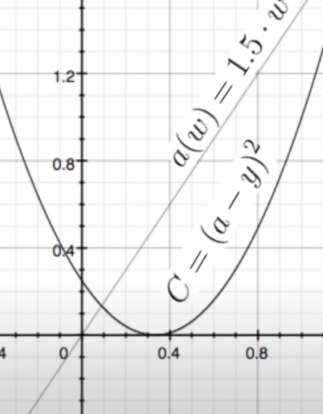we can also see that the minimum value of the error function is also near 0.3

Now, what backpropagation really does is trying to reduce the error sloping downwards the error function. In order to figure out which way is down, it needs to calculate the slope of each given point. Thus, we need to find the derivative of the cost function which simply is :

dC/da = 2(a-y) . The only problem is that we can not change the output in the network, without changing the weight (If you remember we wrote the output as a function of weight a(x) = i * w) and hence we need an expression which gives us the derivative of our cost function with respect to w. In order to do this, we will use the chain rule and so we have:

C(a) = (a-y)^2
a(w) = i*w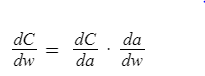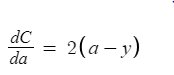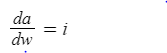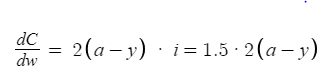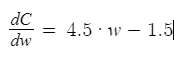Now, we need to update the weight by using a hyperparameter r (learning rate ) which is used to control how much the weight will be changed. In order to update the weight, and find the new one we use the following formula: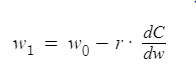Where w1 is the new weight, w0 is the old weight, r is the learning rate. Let’s use r = 0.1. Then: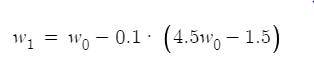Now, if you remember our weight was 0.8 and so the new weight if you do the calculations will be 0.59. But then wait! We had seen in our graph above that the weight must be around 0.3 so we can get our expected result. Have we done anything wrong? The answer is no. If we continue replacing w0 with 0.59 finding the new w1 and continue this process we will have the following results: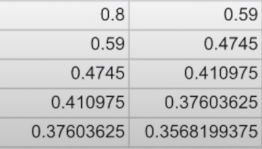As you can see, the weights are going closer to 0.3 and particularly to 0.3333… The speed of getting the best weight depends on the learning rate (which is why it is named with this name)

And this is what Backpropagation really is.

# Conclusion and Credits

I hope that you have now a better understanding of what backpropagation really is. If not, I hope that you are motivated enough by this article to carry out your own research and find out more about it .Maybe in the future , I can try to write an other article that covers backpropagation in more complex neural networks, but the basics(which are what we discussed here) remain the same. The idea and many of the examples of the above explanation were mainly covered in a YouTube video that gave me the idea to write this article, so all credits go to that great channel.

If you want to learn more about backpropagation and the math behind neural networks you can also visit this article.

18 Likes